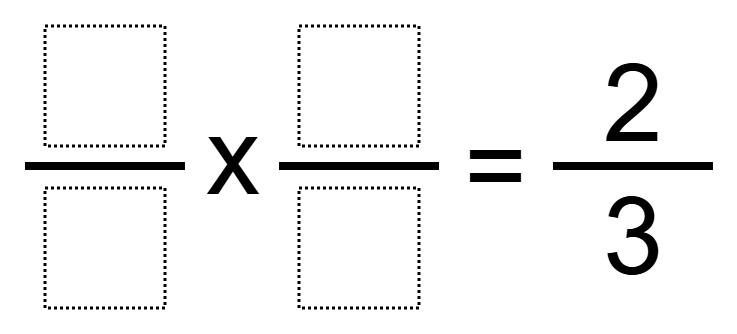# Multiplying Fractions 5

Directions: Using the digits 1 to 9 at most one time each, place a digit in each box to make a true equation.### Hint

How can there be more than one way to make a product of 2/3?

There are many possible answers including 4/3 x 1/2.

Source: Robert Kaplinsky

## Equivalent Expressions with Fractions

Directions: Using the digits 0 to 9 at most one time each and choosing either …

1.•2.3.•